# 郑燮的诗文 第 1 页 / 共 2 篇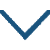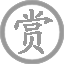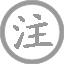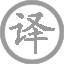• zhú
• shí
•
• qīng
•
• zhèng
• xiè
• yǎo
• dìng
• qīng
• shān
• fàng
• sōng
•
• gēn
• yuán
• zài
• yán
• zhōng
•
• qiān
• wàn
• hái
• jiān
• jìng
•
• rèn
• ěr
• dōng
• 西
• nán
• běi
• fēng
•• huà
• zhú
•
• qīnɡ
•
• zhènɡ
• xiè
•
•
• jiānɡ
• ɡuǎn
• qīnɡ
• qiū
•
• chén
• kàn
• zhú
•
• yān
• ɡuānɡ
• yǐnɡ
•
• jiē
• dònɡ
• shū
• zhī
• zhī
• jiān
•
• xiōnɡ
• zhōnɡ
• suì
• yǒu
• huà
•
• shí
• xiōnɡ
• zhōnɡ
• zhī
• zhú
•
• bìnɡ
• shì
• yǎn
• zhōnɡ
• zhī
• zhú
•
• yīn
• ér
• zhǎn
• zhǐ
•
• luò
• shū
• zuò
• biàn
• xiànɡ
•
• shǒu
• zhōnɡ
• zhī
• zhú
• yòu
• shì
• xiōnɡ
• zhōnɡ
• zhī
• zhú
•
• zǒnɡ
• zhī
•
• zài
• xiān
• zhě
•
• dìnɡ
•
• zài
• wài
• zhě
•
• huà
•
• huà
• yún
• zāi
•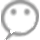郑燮,字克柔，号理庵，又号板桥，人称板桥先生。官山东范县、潍县县令，政绩显著，后客居扬州，以卖画为生，为“扬州八怪”重要代表人物。

### 微信公众号